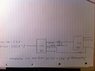# Implementing a boolean function using two 2X1 multiplexers

bd411

## Homework Statement

Implement the following function using two 2 X 1 multiplexers.

F = A'B'C' + AB + AC

Where A' = NOT A;
and A = A.

## Homework Equations

No equations although knowledge of the workings of the multiplexers is required. The top line on the box labelled MUX is the data select line, and selects one of two (hence 2X1) inputs to appear at the output.

## The Attempt at a Solution

I believe I have a solution although pretty much stumbled across it by perseverance. If anyone can tell me a methodical way to determine the solution it would be most appreciated ! Also, I don't know whether my solution is unnecessarily complex ? Any suggestions for simplification are most welcome and appreciated !

#### Attachments

•MUX.jpg
27.2 KB · Views: 1,301

Mentor

## Homework Statement

Implement the following function using two 2 X 1 multiplexers.

F = A'B'C' + AB + AC

Where A' = NOT A;
and A = A.

## Homework Equations

No equations although knowledge of the workings of the multiplexers is required. The top line on the box labelled MUX is the data select line, and selects one of two (hence 2X1) inputs to appear at the output.

## The Attempt at a Solution

I believe I have a solution although pretty much stumbled across it by perseverance. If anyone can tell me a methodical way to determine the solution it would be most appreciated ! Also, I don't know whether my solution is unnecessarily complex ? Any suggestions for simplification are most welcome and appreciated !

Are you supposed to use only 2 MUXs and then you can use whatever other logic you want? That seems a bit strange... Usually you would be asked to use only MUXs and no other logic... (but I think 2 MUXs alone wouldn't be enough on this problem...)

As for a general synthesis technique, I can think of one, but I don't know if it's the most optimal one. With the 2-input MUX you can implement an AND function. With a 2-input MUX you can implement a NOT function. And since you can make any logic function you want using only NAND gates, you should be able to make any logic function with only 2-input MUXs...

haxor489
Yea i also don't think they intended for you to use logic gates as you could express the entire function with logic gates.

Heres what i got but I can't say i used any real process i just thought about it long enough.
Looking at the truth table i noticed that when B was 1 F was A. That took care of half the possible inputs of ABC, looking at the cases of B = 0 i noticed when A was 1 F was C and when A was 0 F was C'.

select lines are bottom inputs

#### Attachments

bd411
Thanks a lot for the help guys !

Yeah I see what you guys mean ... the question (as it was worded) was:

Implement the function using
(a) one 4 X 1 multiplexer - easy enough
(b) two 2 X 1 multiplexers.

Although it doesn't specifically say that I can't use any other logic, I would have marks deducted for an unnecessarily complex solution.

Thanks a lot, I guess the process is to look long and hard at the truth table then !

Mentor
Yea i also don't think they intended for you to use logic gates as you could express the entire function with logic gates.

Heres what i got but I can't say i used any real process i just thought about it long enough.
Looking at the truth table i noticed that when B was 1 F was A. That took care of half the possible inputs of ABC, looking at the cases of B = 0 i noticed when A was 1 F was C and when A was 0 F was C'.

select lines are bottom inputs

Please don't do students' homework for them here on the PF. That's against the PF rules.

haxor489
Sorry Berkeman, didn't know, I'll hint towards solutions next time.